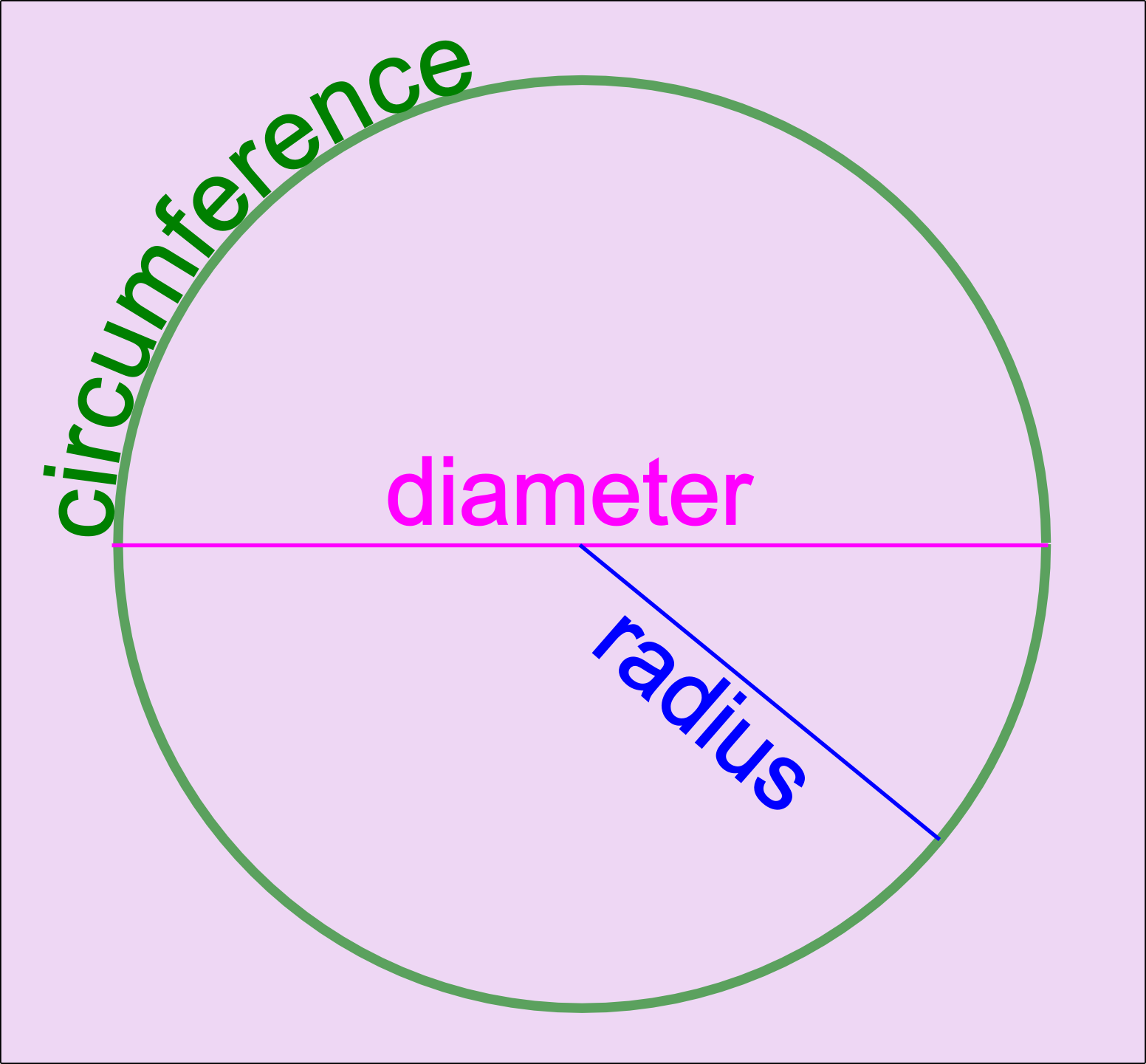The Singing Hedgehog Guide to:
Circlesπ is the Greek letter p or "pi"

In a circle,
π is just how many times the distance across a circle will fit round the outside of the circle, which is a little over three

The normal approximations for
π are 3.14 or 22/7

We have some formulae to remember:
• diameter = twice the radius
• radius = half the diameter
• circumference = pi x diameter
C = πd
• area = pi x radius squared
A = π